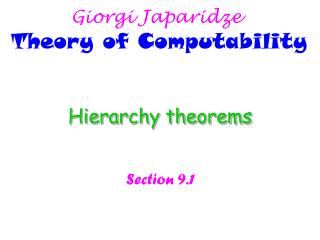# Hierarchy theorems - PowerPoint PPT PresentationDownload PresentationHierarchy theorems

Hierarchy theorems
Download Presentation## Hierarchy theorems

- - - - - - - - - - - - - - - - - - - - - - - - - - - E N D - - - - - - - - - - - - - - - - - - - - - - - - - - -
##### Presentation Transcript

1. Giorgi Japaridze Theory of Computability Hierarchy theorems Section 9.1

2. 9.1.a Giorgi JaparidzeTheory of Computability Space constructibility Definition 9.1 A function f: NN, where f(n) is at least O(log n), is called space constructible if the function that maps any string w of length n (equivalently, the string 1n) to the binary representation of the number f(n) is computable in space O(f(n)). Intuition: Assume a machine M runs in f(n) space, and f(n) is space constructible. Then, for any input w of size n, using only O(f(n)) space, we can not only tell whether M accepts w, but can also compute the value of f(n) itself, i.e. the amount of space used by M on input w. Most natural functions are space constructible. Those that are not are “pathological” cases --- cases where computing the space bound is so expensive that its space cost exceeds the bound itself. This is like if counting money was more expensive than the amount of money itself. Motivation: When f(n) is space constructible, we can easily construct machines for whatever purposes that control their own space consumption and make sure that it does not exceed f(n).

3. 9.1.b Giorgi JaparidzeTheory of Computability Space hierarchy theorem Theorem 9.3 For any space constructible function f: NN, a language A exists that is decidable in O(f(n)) space but not in o(f(n)) space. Proof. Such a language A is the one decided by the following algorithm (TM): D = “On input w: 1. Let n be the length of w. 2. Compute f(n) using space constructibility, and mark off this much tape. If later stages ever attempt to use more, reject. 3. If w is not of the form <M>10* for some TM M, reject. 4. Simulate M on w while counting the number of steps used in the simulation. If the count ever exceeds 2f(n), reject. 5. If M accepts, reject. If M rejects, accept.” Step 4 guarantees that D is indeed a decider (why?). Step 2 guarantees that D runs in space O(f(n)) (why?). Step 5 guarantees that A is different from the language decided by any o(f(n)) space machine (why?).

4. 9.1.c Giorgi JaparidzeTheory of Computability Below and elsewhere “AB” means “AB and A≠B”. Corollaries of the space hierarchy theorem EXPSPACE is defined as SPACE(2n1)  SPACE(2n2)  SPACE(2n3)  … Corollary 9.4 For any two functions f1,f2: N N, where f1 is o(f2(n)) and f2is space constructible, SPACE(f1(n))  SPACE(f2(n)). Corollary 9.5 For any two real numbers 0 ≤1< 2, SPACE(n1)  SPACE(n2). Corollary 9.6NL  PSPACE. Proof. This is so because NLSPACE(log2n) (by Savitch’s theorem), and SPACE(log2n)SPACE(n) (by the space hierarchy theorem). Corollary 9.7PSPACE  EXPSPACE.

5. 9.1.d Giorgi JaparidzeTheory of Computability Time hierarchy theorem Definition 9.8 A function t: NN, where t(n) is at least O(n log n), is called time constructible if the function that maps any string w of length n to the binary representation of the number t(n) is computable in time O(t(n)). Theorem 9.10 For any time constructible function t: NN, a language A exists that is decidable in O(t(n)) time but not in time o(t(n)/log t(n)). Proof. Such an A is the one decided by the following O(t(n)) time algorithm (why?): D = “On input w: 1. Let n be the length of w. 2. Compute t(n) using time constructibility, and store the value t(n)/log t(n) (roun- ded up to the nearest integer) in a binary counter. Decrement this counter before each step used to carry out stages 3, 4 and 5. If the counter ever hits 0, reject. 3. If w is not of the form <M>10* for some TM M, reject. 4. Simulate M on w. 5. If M accepts, reject. If M rejects, accept.”

6. 9.1.e Giorgi JaparidzeTheory of Computability Corollaries of the time hierarchy theorem Corollary 9.11 For any two functions t1,t2: N N, where t1 is o(t2(n)/log t2(n)) and t2is time constructible, TIME(t1(n))  TIME(t2(n)). Corollary 9.12 For any two real numbers 0 ≤1< 2, TIME(n1)  TIME(n2). Corollary 9.13P  EXPTIME.

7. 9.1.f Giorgi JaparidzeTheory of Computability EXPSPACE-completeness Definition 9.14 A language B is EXPSPACE-complete if 1. B is in EXPSPACE, and 2. every language A in EXPSPACE is polynomial time reducible to B. • Regular expressions with exponentiation are defined in the same was as (ordinary) • regular expressions, but with the additional formation rule: • If E is a regular expression with exponentiation and k is a decimal number, then • Ek is also a regular expression with exponentiation. • The meaning of Ek is E concatenated with itself k times. • Examples: 024 000 000(171*); 018000 00006000 0001* EQREX = {<Q,R> | Q and R are equivalent regular expressions with exponentiation}. Theorem 9.15 EQREX is EXPSPACE-complete. Proof omitted.